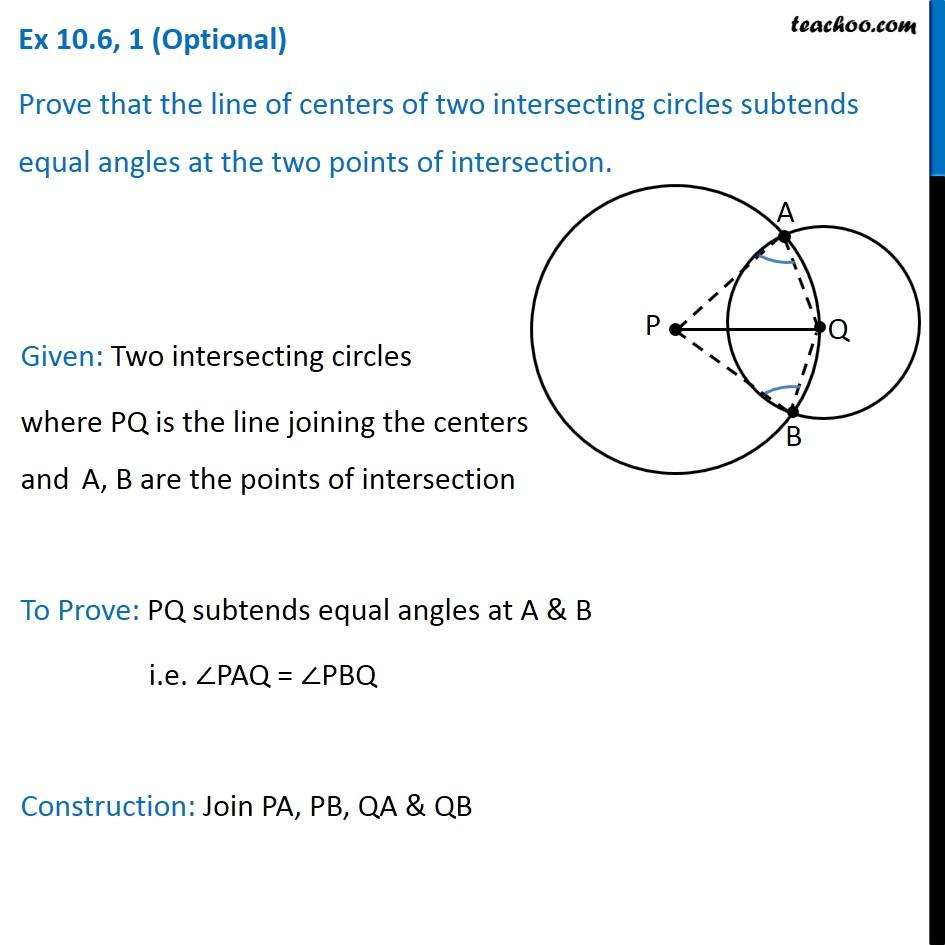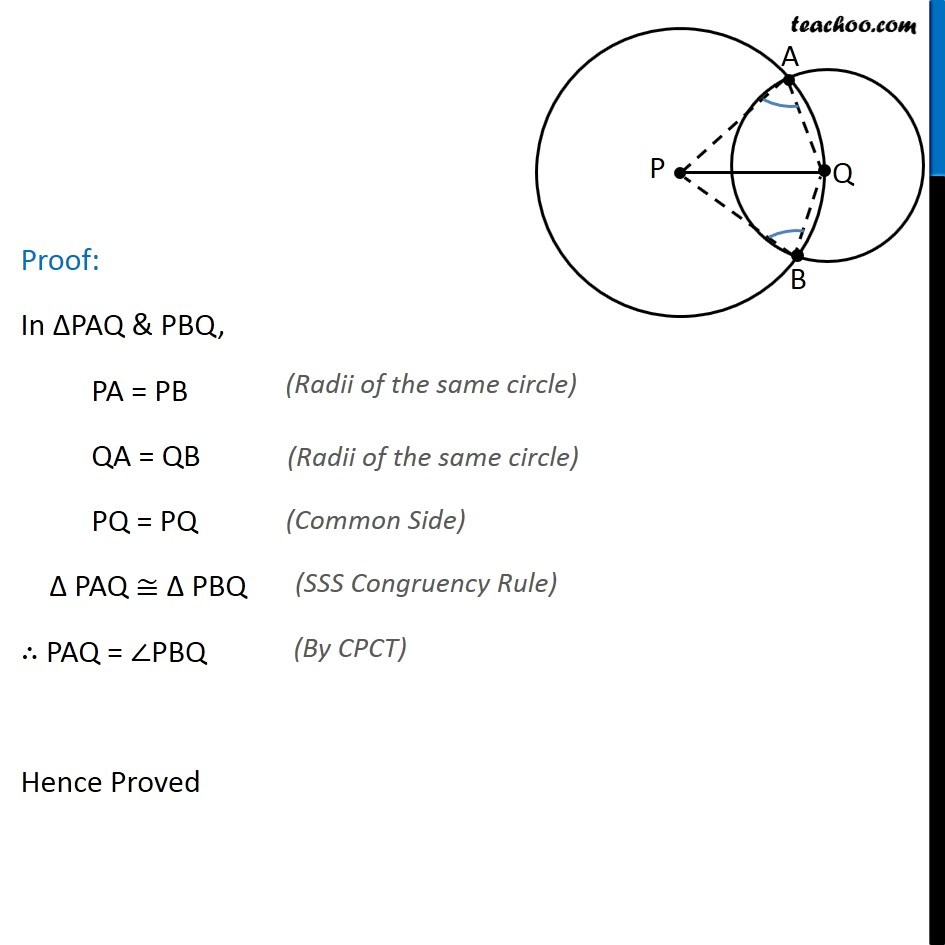Important Questions on Circles

Chapter 9 Class 9 Circles
Serial order wiseLearn in your speed, with individual attention - Teachoo Maths 1-on-1 Class

### Transcript

Question 1 Prove that the line of centers of two intersecting circles subtends equal angles at the two points of intersection. Given: Two intersecting circles where PQ is the line joining the centers and A, B are the points of intersection To Prove: PQ subtends equal angles at A & B i.e. ∠PAQ = ∠PBQ Construction: Join PA, PB, QA & QB Proof: In ∆PAQ & PBQ, PA = PB QA = QB PQ = PQ ∆ PAQ ≅ ∆ PBQ ∴ PAQ = ∠PBQ Hence Proved (Radii of the same circle) (Radii of the same circle) (Common Side) (SSS Congruency Rule) (By CPCT)